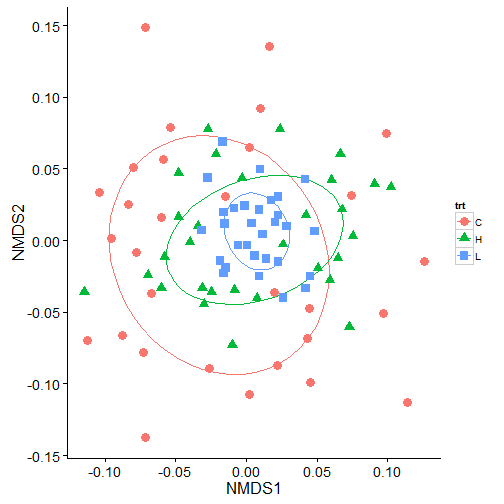# Permutational multivariate analysis of variance using distance matrices (adonis)

The RMarkdown source to this file can be found here

Wow! I did not realize that it has been a full three months since I have last done a post on here.

I have done several posts on how to plot several different processes with ggplot2 and this one will yet again fall into this category. Back in April I posted about how to plot NMDS plots from the vegan package in ggplot2. Another powerful function in the vegan package, is `adonis()`. `adonis` allows you to do permutational multivariate analysis of variance using distance matrices.

Recently, a graduate student recently asked me why `adonis()` was giving significant results between factors even though, when looking at the NMDS plot, there was little indication of strong differences in the confidence ellipses. So I thought I would create a little post illustrating what adonis is partly doing and how to visually represent what was being done in the analysis, in hopes to illustrate why significant differences were found.

### Creating the data

First lets create some data. We will create three sets of sites (30 sites, 10 species) for each of three treatments. The number of individuals for each species in a site will be drawn from a negative binomial distribution using `rnbinom()` using the a similar mean number of species but allowing the dispersion parameter to be different. Note: This data was created just to illustrate this post and I am sure it could be done better to illustrate actual ecological data and provide better NMDS fits.

### Running an NMDS

Then we can run this through `metaMDS` and plot it in `ggplot` using `stat_ellipse` to generate the confidence ellipses.In the above plot, we can see a lot of overlap in the 50% ellipses and the centroids are not that different suggesting that the groups are not that different. But, running the same data in `adonis` indicates that there are significant differences in the treatments.

So why do we get a significant value from adonis? `adonis` works by first finding the centroids for each group and then calculates the squared deviations of each of site to that centroid. Then significance tests are performed using F-tests based on sequential sums of squares from permutations of the raw data.

A good way to see why we are getting differences by plotting this out. The process is to calculate this distance matrix for the data using the `vegdist` function and then calculate the multivariate homogeneity of group dispersions (variances) using `betadisper`. For more information on the process behind this read the Details from `help(betadisper)`.

### Visualizing the multivariate homogeneity of group dispersions

We can then plot this out in steps so it is easier to visualize. First, I will extract the data and get it in a forma that ggplot2 can use.

I will use `grid.arrange` from gridExtra to create display each treatment seperately and then have a combined panel.

First points (black symbols) and the centroids (red symbols).Then the vector segmentsThen the hullsIn the above data, we can see that the control data has the greatest variance (i.e., differences between each black point and the red centroid) in the data, followed by the high treatment, and then the low treatment. The significance shown by adonis, in the case of this data, is due to the variation associated with the treatment groups. This should not surprising given that when we created data at the beginning, we used the same mean number of individuals and just differed the size argument in `rnbinom()`.

Tags:

Categories:

Updated: